• 二、基础篇： 围绕Python基础语法介绍、数据预处理、数据可视化以及数据分析与挖掘......这些核心技能模块展开，帮助你快速而全面的掌握和了解成为一个数据分析师的所有必修功法。   三、数据采集篇： 通过...python工程师 python爬虫 python python教程 python安装
• Python数据分析与挖掘实战第三章 数据探索3.1 数据质量分析3.1.1 缺失值分析3.1.2 异常值分析3.2 数据特征分析3.2.1 统计量分析3.2.2 贡献度分析3.2.2 相关性分析 第三章 数据探索 3.1 数据质量分析 3.1.1 缺失值...
Python数据分析与挖掘实战第三章 数据探索3.1 数据质量分析3.1.1 缺失值分析3.1.2 异常值分析3.2 数据特征分析3.2.1 统计量分析3.2.2 贡献度分析3.2.3 相关性分析3.2.4 统计特征函数3.2.5 绘图函数第四章 数据预处理4.1 数据清洗4.1.1 缺失值处理4.2 数据变换4.1.1 数据归一化4.1.2 数据离散化(聚类)4.3 属性改造4.4 数据规约4.5 常用预处理函数第五章 挖掘建模
第三章 数据探索
3.1 数据质量分析
3.1.1 缺失值分析
缺失值的处理分为三种情况:

删除存在缺失值的记录;
对可能的数据进行插值:拉格朗日插值,牛顿插值法:

3.1.2 异常值分析
首先可以先使用describe()函数查看数据的基本情况:
import pandas as pd
# 餐饮数据
catering_sale = './data/catering_sale.xls'
# 读取数据，指定日期列为索引列
data.describe()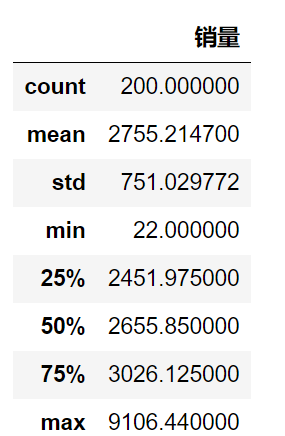检测异常值的方法可以使用箱型图:
import pandas as pd
catering_sale = './data/catering_sale.xls'
import matplotlib.pyplot as plt
# 用来正常显示中文标签
# plt.rcParams['dont.sans-serif'] = ['SimHei']
plt.rcParams['font.sans-serif'] = ['SimHei']    # 用来正常显示中文标签
# # 用来正常显示负号
plt.rcParams['axes.unicode_minus'] = False
plt.figure()
'''
画箱型图，这里画箱型图有两种方法:
1. 一种是直接调用DataFrame的boxplot()；
2. 另一种是调用Series或者DataFrame的plot()方法,并用kind参数指定箱型图(box);
'''
p = data.boxplot(return_type='dict')
# 'flies'即为异常值的标签
x = p['fliers'].get_xdata()
y = p['fliers'].get_ydata()
y.sort()
for i in range(len(x)):
if i > 0:
plt.annotate(y[i],xy = (x[i],y[i]), xytext = (x[i]+0.05-0.8/(y[i]-y[i-1]),y[i]))
else:
plt.annotate(y[i],xy = (x[i],y[i]),xytext = (x[i]+0.08,y[i]))
plt.show()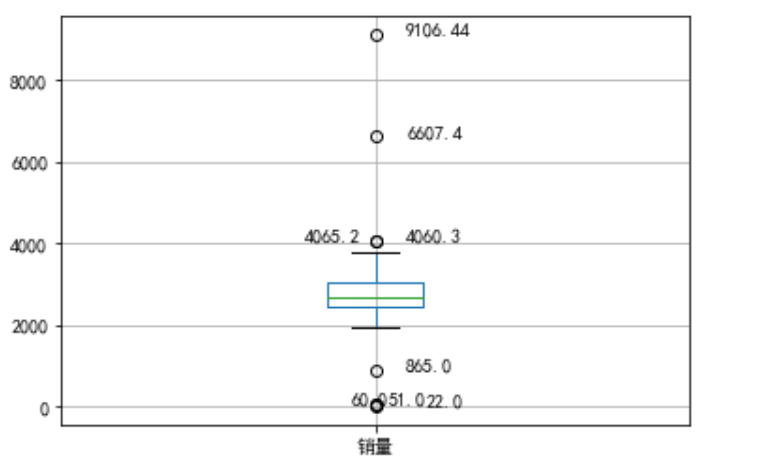上下的两个标线表示的是上界和下界(四分位点),超过上下界的值就是异常,但是那其中几个散点离上下界比较近,所以可以把865,4060.3,4065.2归为正常值,将22,51,60,6607.4,9106.44归为异常值;
3.2 数据特征分析
对于定量数据可以通过绘制频率分布表,绘制频率分布直方图,茎叶图的方式进行直观的分析;
对于定性分类的数据,可以使用饼图和条形图的方式来查看显示分布情况;
3.2.1 统计量分析
极差反映了最大值和最小值的分布情况;
标准差用来度量数据偏离均值的程度;
变异系数度量标准差相对于均值的离中趋势;
四分位数间距表示上下四分位数之差,越大表示变异程度越大;
# 餐饮销量数据统计量分析
import pandas as pd
catering_sale = './data/catering_sale.xls'
# 过滤异常数据
data = data[(data[u'销量'] > 400)&(data[u'销量'] < 5000)]
statistics = data.describe()
# 极差
statistics.loc['range'] = statistics.loc['max'] - statistics.loc['min']
# 变异系数
statistics.loc['var'] = statistics.loc['std'] / statistics.loc['mean']
# 四分位数间距
statistics.loc['dis'] = statistics.loc['75%'] - statistics.loc['25%']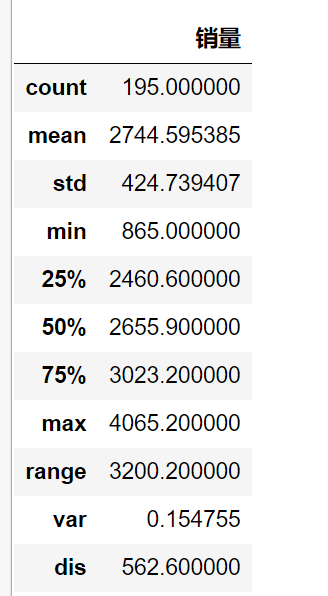3.2.2 贡献度分析
# 菜品盈利帕累托图代码
import pandas as pd
# 初始化参数
dish_profit = './data/catering_dish_profit.xls'
data = data[u'盈利'].copy()
# 表示按降序排列
data.sort_values(ascending=False)
# 导入图像库
import matplotlib.pyplot as plt
plt.rcParams['font.sans-serif'] = ['SimHei']    # 用来正常显示中文标签
plt.rcParams['axes.unicode_minus'] = False

plt.figure()
# 显示直方图
data.plot(kind='bar')
plt.ylabel(u'盈利(元)')
p = 1.0 * data.cumsum()/data.sum()
p.plot(color = 'r',secondary_y=True,style='-o',linewidth=2)
# 添加注释，即85%处的标记，这里包括了指定箭头样式
plt.annotate(format(p,'.4%'),xy=(6,p),xytext=(6*0.9,p*0.9),
plt.ylabel(u'盈利')
plt.show()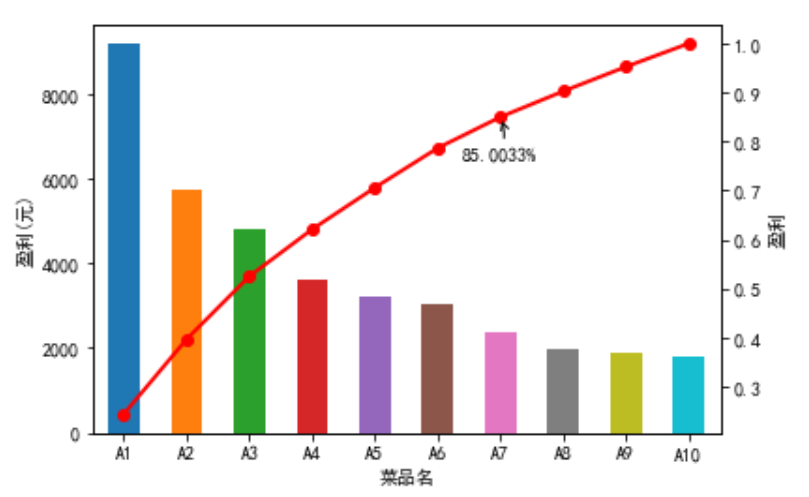3.2.3 相关性分析
import pandas as pd
# 餐饮数据，含有其他属性
catering_sale = './data/catering_sale_all.xls'
# 读取数据，指定'日期'列为索引列
# 相关系数矩阵,即给出了任意两款菜式之间的相关系数
data.corr()
# 只显示"百合酱蒸风爪"与其他菜式的相关系数
data.corr()[u'百合酱蒸凤爪']
# 计算两者的相关系数
data[u'百合酱蒸凤爪'].corr(data[u'翡翠蒸香茜饺'])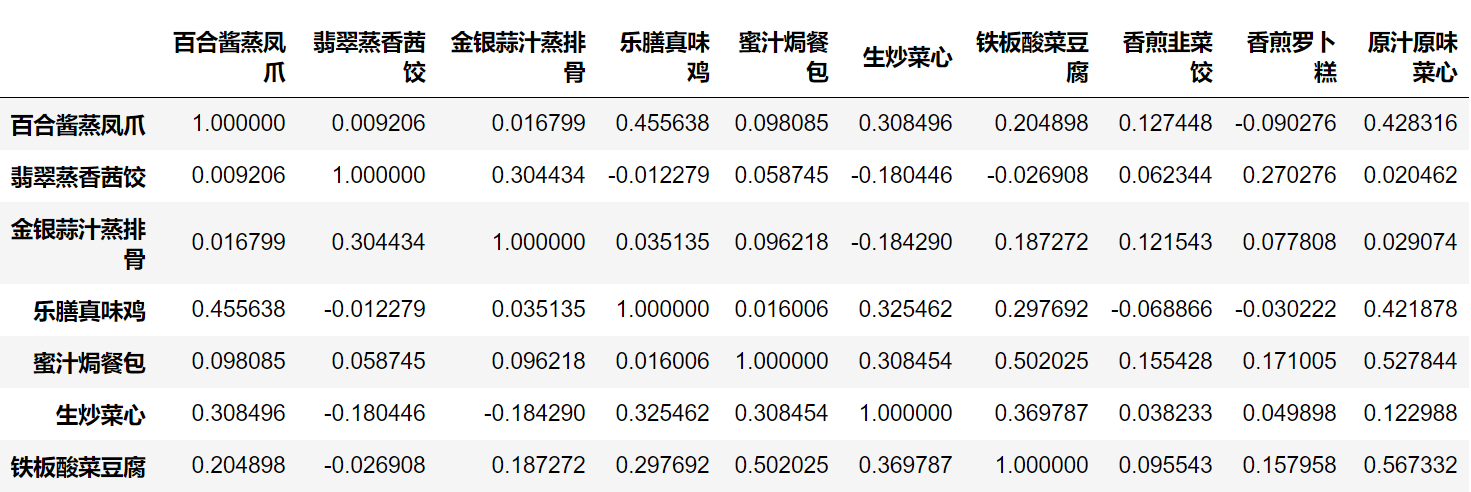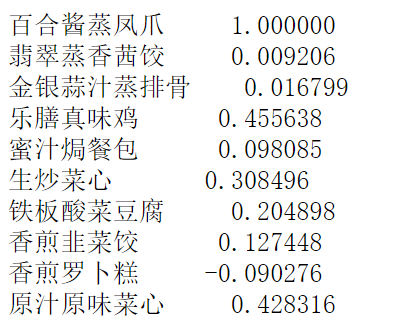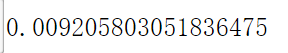计算两个列向量的相关系数:
# 计算两个列的相关系数
# 生成样本D,一行为1-7，一行为2-8
D = pd.DataFrame([range(1,8),range(2,9)])
# 提取第一行
S1 = D.loc
# 提取第二行
S2 = D.loc
# 计算S1，S2的相关系数:有person(皮尔逊系数),kendall(肯德尔系数),spearman(斯皮尔曼系数)
S1.corr(S2,method='pearson')

计算协方差矩阵:
# 计算协方差矩阵
import numpy as np
D = pd.DataFrame(np.random.randn(6,5)) # 产生6*5的随机矩阵
D.cov() #计算协方差矩阵
D.cov(D)  # 计算第一列和第二列的协方差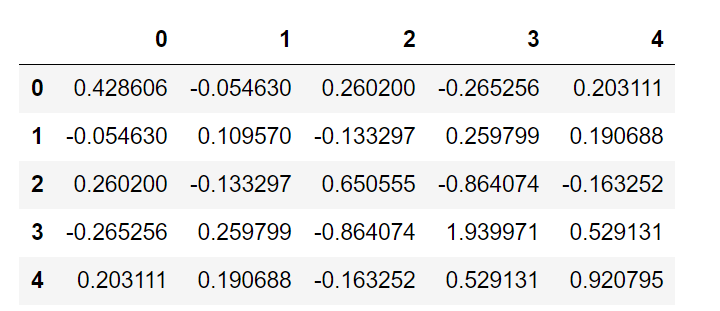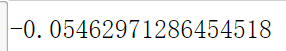计算 6 * 5 随机矩阵的偏度(三阶矩)/峰度(四阶矩)
# 计算 6 * 5 随机矩阵的偏度(三阶矩)/峰度(四阶矩)
import pandas as pd
D = pd.DataFrame(np.random.randn(6,5)) # 产生6*5的随机矩阵
# 偏度:通过对偏度系数的测量，我们能够判定数据分布的不对称程度以及方向
D.skew()
# 峰度:是研究数据分布陡峭或平滑的统计量，通过对峰度系数的测量，我们能够判定数据分布相对于正态分布而言是更陡峭还是平缓。
D.kurt()

3.2.4 统计特征函数
使用cumsum来获取某一列的前n项和:
# 使用cumsum来获取某一列的前n项和
D = pd.Series(range(0,20)) # 构造Series，内容为0-19共20个整数
D.cumsum(0) # 给出前n项和
# 问题：当其大于列数时，表示什么意思呢？

3.2.5 绘图函数
直线:
import matplotlib.pyplot as plt # 导入作图库
plt.rcParams['font.sans-serif'] = ['SimHei'] # 用来正常显示中文标签
plt.rcParams['axes.unicode_minus'] = False # 用来正常显示负号
plt.figure(figsize = (7,5)) # 创建图像区域，指定比例
import numpy as np
#设置起点,终点和步长
x = np.linspace(0, 2*np.pi, 50)  # x坐标输入
y = np.sin(x) # 计算对应x的正弦值
plt.plot(x, y, 'bp--') # 控制图形格式为蓝色带星虚线，显示正弦曲线
plt.show()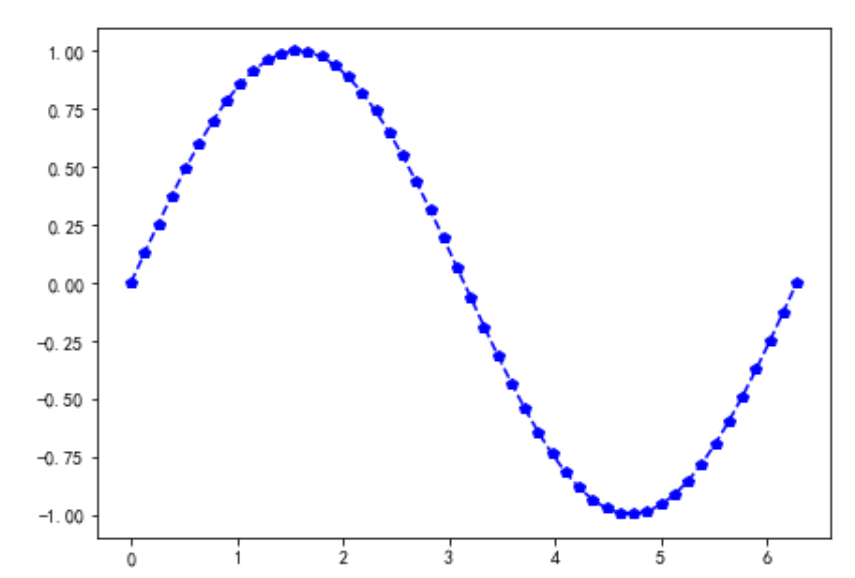饼图:
import matplotlib.pyplot as plt
labels = 'Frogs','Hogs','Dogs','Logs' # 定义标签
sizes = [15, 30, 45, 10] #每一块的比例
colors = ['yellowgreen', 'gold', 'lightskyblue', 'lightcoral'] #每一块的颜色
explode = (0,0.09,0,0) # 突出显示，这里仅仅突出第二块
plt.pie(sizes,explode=explode, labels=labels, colors=colors, autopct='%1.1f%%',
plt.axis('equal') #显示为圆(避免比例压缩为椭圆)
plt.show()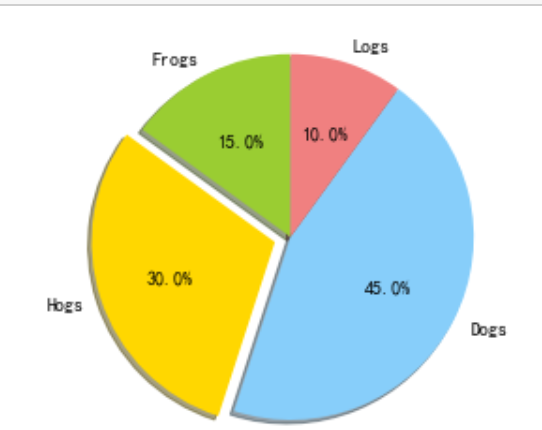直方图:
import matplotlib.pyplot as plt
import numpy as np
x = np.random.randn(1000) # 1000个服从正态分布的随机数
plt.hist(x, 10) # 分成10组进行绘制直方图
plt.show()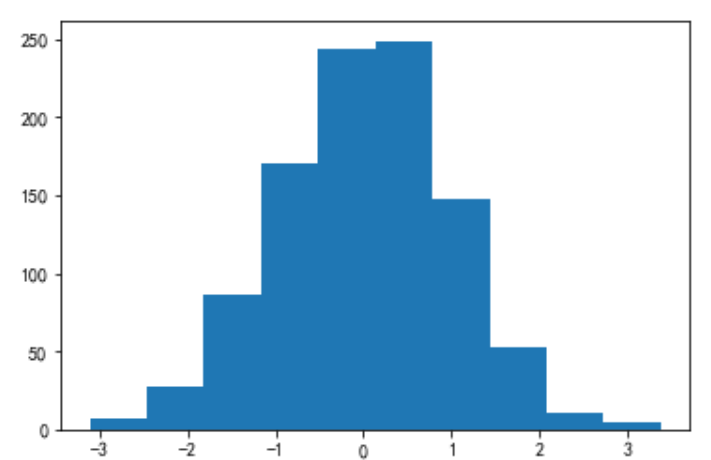箱型图
'''
绘制箱型图的两种方法:
1. 直接调用DataFrame的boxplot()方法；
2. 调用Series或者DataFrame的plot()方法,并用kind参数指定箱型图(box);
'''
import matplotlib.pyplot as plt
import numpy as np
import pandas as pd
x = np.random.randn(1000) # 1000个服从正态分布的随机数
D = pd.DataFrame([x,x+1]).T # 构造两列的DataFrame
D.plot(kind = 'box') # 调用Series内置的作图方法画图，用kind参数指定箱型图box
plt.show()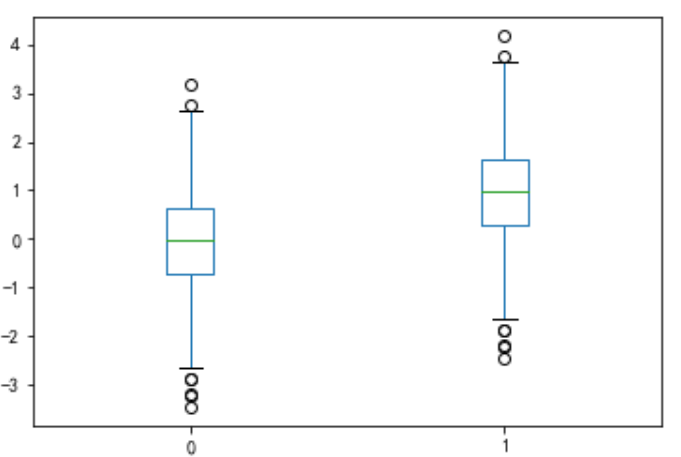折线图:
import matplotlib.pyplot as plt
plt.rcParams['font.sans-serif'] = ['SimHei'] # 用来正常显示中文标签
plt.rcParams['axes.unicode_minus'] = False # 用来正常显示负号

import numpy as np
import pandas as pd
# np.arrange()返回一个ndarray而不是一个列表
x = pd.Series(np.exp(np.arange(20))) # 原始数据
# 对图像左上方做好标记
x.plot(label = u'原始数据图',legend = True)
plt.show()
x.plot(logy = True, label = u'对数数据图',legend = True)
plt.show()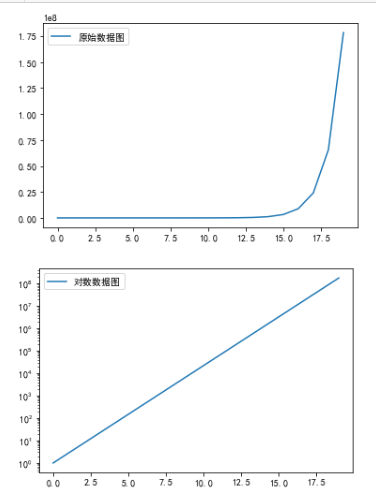import matplotlib.pyplot as plt
plt.rcParams['font.sans-serif'] = ['SimHei'] # 用来正常显示中文标签
plt.rcParams['axes.unicode_minus'] = False # 用来正常显示负号

import numpy as np
import pandas as pd
error = np.random.randn(10) # 定义误差列
y = pd.Series(np.sin(np.arange(10))) #均值数据列
y.plot(yerr = error) #绘制误差图
plt.show()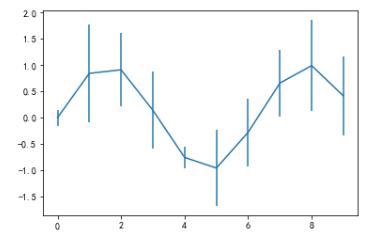第四章 数据预处理
4.1 数据清洗
4.1.1 缺失值处理
缺失值处理一般采用:均值/中位数/众数插补,使用固定值,最近邻值插补,回归法,插值法
拉格朗日插值:
# 用拉格朗日法进行插补
import pandas as pd
from scipy.interpolate import lagrange # 导入拉格朗日函数
import xlwt
inputfile = './data/catering_sale.xls' # 销售数据路径
outputfile = './sales.xls' # 输出数据路径
data[u'销量'][(data[u'销量'] < 400) | (data[u'销量'] > 5000)] = None # 过滤异常值，将其变为空值

# 自定义列向量插值函数
# s为列向量，n为被插值的位置，k为取前后的数据个数，默认为5
def ployinterp_column(s, n, k=5):
y = s[list(range(n-k,n)) + list(range(n+1, n+1+k))] # 取数
y = y[y.notnull()] # 剔除空值
return lagrange(y.index, list(y))(n) # 插值并返回插值结果

# 逐个元素判断是否需要插值
for i in data.columns:
for j in range(len(data)):
if (data[i].isnull())[j]: #如果为空即插值
data[i][j] = ployinterp_column(data[i],j)

data.to_excel(outputfile) # 输出结果, 写入文件

4.2 数据变换
4.1.1 数据归一化
# 数据规范化
import pandas as pd
import numpy as np
datafile = './data/normalization_data.xls' #参数初始化
(data - data.min())/(data.max() - data.min()) # 最小-最大规范化
(data - data.mean())/data.std() # 零-均值规范化
data/10**np.ceil(np.log10(data.abs().max())) # 小数定标规范化

4.1.2 数据离散化(聚类)
等宽法,等频法,基于聚类分析的方法;
def cluster_plot(d, k):  # 自定义作图函数来显示聚类结果
import matplotlib.pyplot as plt
plt.rcParams['font.sans-serif'] = ['SimHei'] # 用来正常显示中文标签
plt.rcParams['axes.unicode_minus'] = False # 用来正常显示负号
plt.figure(figsize = (8, 3))
for j in range(0, k):
plt.plot(data[d==j], [j for i in d[d==j]], 'o')
plt.ylim(-0.5, k-0.5)
return plt

# 数据离散化:根据某列的数值来进行分组
import pandas as pd
datafile = './data/discretization_data.xls' #参数初始化
data = data[u'肝气郁结证型系数'].copy()
k = 4
# 1. 等宽离散化,各个类别依次命名为0,1,2,3
d1 = pd.cut(data, k, labels= range(k))
# 2. 等频率离散化
w = [1.0*i/k for i in range(k+1)]
w = data.describe(percentiles = w)[4:4+k+1] # 使用describe函数自动计算分位数
w = w * (1-1e-10)
d2 = pd.cut(data, w, labels = range(k))

# 3. 基于聚类分析的方法
from sklearn.cluster import KMeans # 引入KMeans
kmodel = KMeans(n_clusters = k, n_jobs = 4) # 建立模型,n_jobs是并行数,一般等于CPU数较好
kmodel.fit(data.values.reshape((len(data), 1)))
c = pd.DataFrame(kmodel.cluster_centers_).sort_values(by=0) # 输出聚类中心,并且排序(默认随机排序)

# # python3中没有rolling_mean的方法,需要将其改为rolling.mean
w = c.rolling(2).mean().iloc[1:]
# w
# .mean(2).iloc[1:] # 相邻两项求中点,作为边界点
w =  + list(w) + [data.max()] # 把首末边界点加上
d3 = pd.cut(data, w, labels = range(k))

cluster_plot(d1, k).show()
cluster_plot(d2, k).show()
cluster_plot(d3, k).show()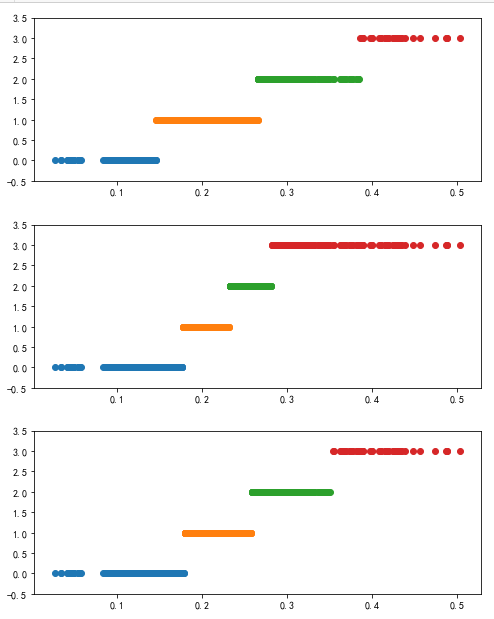4.3 属性改造
# 线损率属性改造
import pandas as pd
# 参数初始化
inputfile = './data/electricity_data.xls' #供入供出电量数据
outputfile = './electricity_data.xls' # 属性改造后的电量数据
data[u'线损率'] = (data[u'供入电量'] - data[u'供出电量'])/data[u'供入电量']
data.to_excel(outputfile, index = False) # 保存结果

4.4 数据规约
# 主成分分析降维代码
inputfile = './data/principal_component.xls'
outputfile = './dimention_reducted.xls' # 降维后的数据
from sklearn.decomposition import PCA
pca = PCA()
pca.fit(data)
pca.components_ # 返回模型的各个特征向量
pca.explained_variance_ratio_ # 返回各个成分各自的方差百分比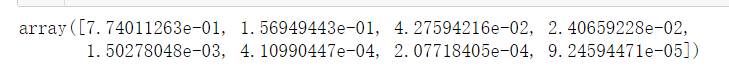# 使用pca的结果
pca = PCA(3)
pca.fit(data)
low_d = pca.transform(data) # 用它来降低维度
pd.DataFrame(low_d).to_excel(outputfile) # 保存结果
pca.inverse_transform(low_d) # 必要时可以用inverse_transform()函数来复原数据

4.5 常用预处理函数
D = pd.Series([1,1,2,3,5])
# 进行数据去重的两种方法
D.unique()
np.unique(D)

第五章 挖掘建模


展开全文• Python数据分析与挖掘之收入的预测分析
Python数据分析与挖掘之收入的预测分析
数据集形式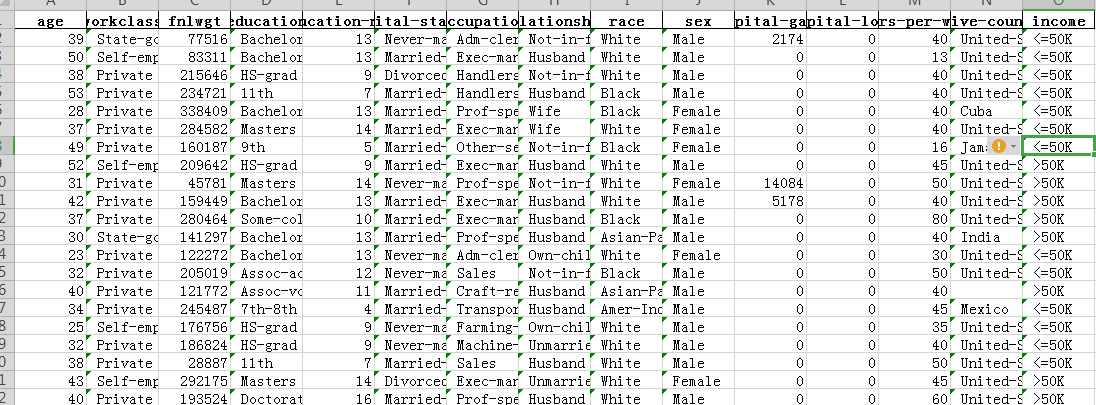# 导入第三方包
import pandas as pd
import numpy as np
import seaborn as sns
# 导入绘图模块
import matplotlib.pyplot as plt
# 导入模型评估模块
from sklearn import metrics
# 导入网格搜索法的函数
from sklearn.model_selection import GridSearchCV
# 数据读取

# 查看数据集是否存在缺失值
print(income.apply(lambda x:np.sum(x.isnull())))

在此处可见workclass，occupation，native-country处值有缺失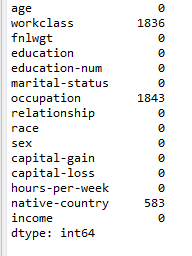缺失值处理
删除法：若确实比例非常小删除法较为合理。
替换法：若缺失为离散型考虑用众数替换，数值型，则考虑用均值或中位数替换缺失值
插补法：基于未缺失的变量预测缺失变量的值，如常见的回归插补法，多重插补法，拉格朗日插补法等
此处用众数进行替换
income.fillna(value = {'workclass':income.workclass.mode(),
'occupation':income.occupation.mode(),
'native-country':income['native-country'].mode()}, inplace = True)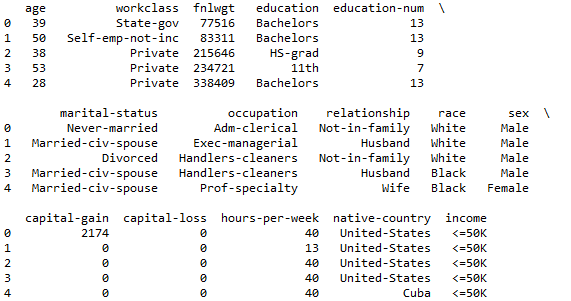# 数据的探索性分析、数值型的统计描述
print(income.describe())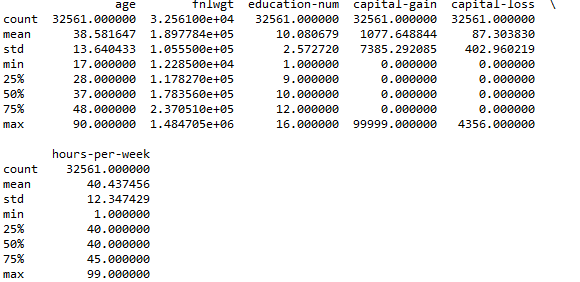# 数据的探索性分析、离散型的统计描述
print(income.describe(include =[ 'object']))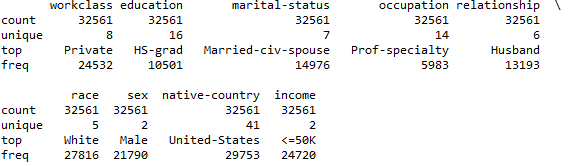# 设置绘图风格
plt.style.use('ggplot')
# 设置多图形的组合
fig, axes = plt.subplots(2, 1)
# 绘制不同收入水平下的年龄核密度图，    针对数值型
income['age'][income.income == ' <=50K'].plot(kind = 'kde', label = '<=50K', ax = axes, legend = True, linestyle = '-')
income['age'][income.income == ' >50K'].plot(kind = 'kde', label = '>50K', ax = axes, legend = True, linestyle = '--')
# 绘制不同收入水平下的周工作小时数和密度图
income['hours-per-week'][income.income == ' <=50K'].plot(kind = 'kde', label = '<=50K', ax = axes, legend = True, linestyle = '-')
income['hours-per-week'][income.income == ' >50K'].plot(kind = 'kde', label = '>50K', ax = axes, legend = True, linestyle = '--')
# 显示图形
plt.show()

此图是描绘仅以被调查居民的年龄和每周工作小时为例，绘制各自的分布形状图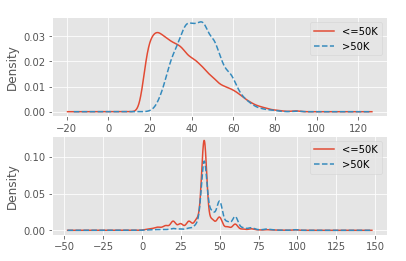# 构造不同收入水平下各种族人数的数据    针对离散型
race = pd.DataFrame(income.groupby(by = ['race','income']).aggregate(np.size).loc[:,'age'])
# 重设行索引
race = race.reset_index()
# 变量重命名
race.rename(columns={'age':'counts'}, inplace=True)
# 排序
race.sort_values(by = ['race','counts'], ascending=False, inplace=True)

# 构造不同收入水平下各家庭关系人数的数据
relationship = pd.DataFrame(income.groupby(by = ['relationship','income']).aggregate(np.size).loc[:,'age'])
relationship = relationship.reset_index()
relationship.rename(columns={'age':'counts'}, inplace=True)
relationship.sort_values(by = ['relationship','counts'], ascending=False, inplace=True)

# 设置图框比例，并绘图
plt.figure(figsize=(9,5))
sns.barplot(x="race", y="counts", hue = 'income', data=race)
plt.show()

plt.figure(figsize=(9,5))
sns.barplot(x="relationship", y="counts", hue = 'income', data=relationship)
plt.show()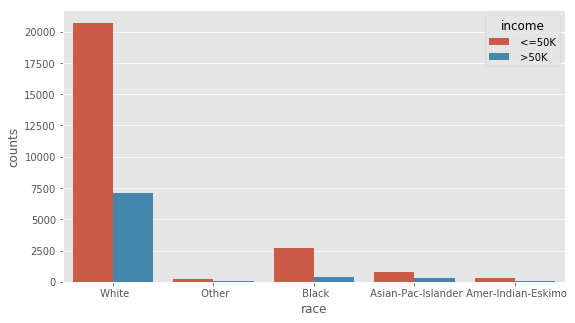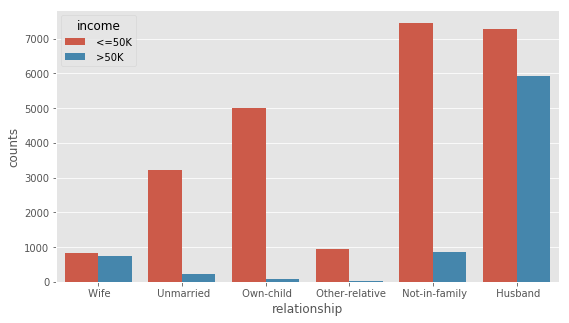# 离散变量的重编码
for feature in income.columns:
if income[feature].dtype == 'object':
income[feature] = pd.Categorical(income[feature]).codes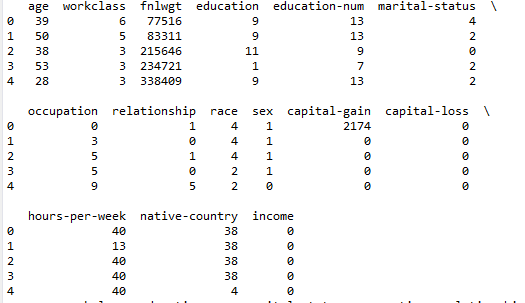# 删除变量
income.drop(['education','fnlwgt'], axis = 1, inplace = True)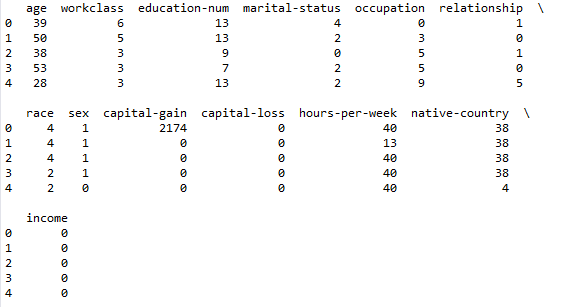# 数据拆分
from sklearn.model_selection import train_test_split

X_train, X_test, y_train, y_test = train_test_split(income.loc[:,'age':'native-country'],
income['income'], train_size = 0.75,
random_state = 1234)
print('训练数据集共有%d条观测' %X_train.shape)
print('测试数据集共有%d条观测' %X_test.shape)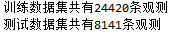# 导入k近邻模型的类
from sklearn.neighbors import KNeighborsClassifier
# 构建k近邻模型
kn = KNeighborsClassifier()
kn.fit(X_train, y_train)
print(kn)

# 预测测试集
kn_pred = kn.predict(X_test)
print(pd.crosstab(kn_pred, y_test))

# 模型得分
print('模型在训练集上的准确率%f' %kn.score(X_train,y_train))
print('模型在测试集上的准确率%f' %kn.score(X_test,y_test))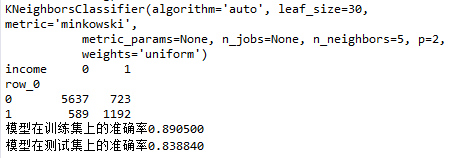# 计算ROC曲线的x轴和y轴数据
fpr, tpr, _ = metrics.roc_curve(y_test, kn.predict_proba(X_test)[:,1])
# 绘制ROC曲线
plt.plot(fpr, tpr, linestyle = 'solid', color = 'red')
# 添加阴影
plt.stackplot(fpr, tpr, color = 'steelblue')
# 绘制参考线
plt.plot([0,1],[0,1], linestyle = 'dashed', color = 'black')
# 往图中添加文本
plt.text(0.6,0.4,'AUC=%.3f' % metrics.auc(fpr,tpr), fontdict = dict(size = 18))
plt.show()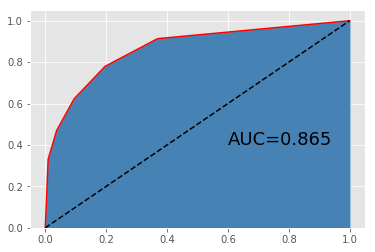# 导入GBDT模型的类
# 构建GBDT模型
gbdt.fit(X_train, y_train)
print(gbdt)

# 预测测试集
gbdt_pred = gbdt.predict(X_test)
print(pd.crosstab(gbdt_pred, y_test))

# 模型得分
print('模型在训练集上的准确率%f' %gbdt.score(X_train,y_train))
print('模型在测试集上的准确率%f' %gbdt.score(X_test,y_test))

# 绘制ROC曲线
fpr, tpr, _ = metrics.roc_curve(y_test, gbdt.predict_proba(X_test)[:,1])
plt.plot(fpr, tpr, linestyle = 'solid', color = 'red')
plt.stackplot(fpr, tpr, color = 'steelblue')
plt.plot([0,1],[0,1], linestyle = 'dashed', color = 'black')
plt.text(0.6,0.4,'AUC=%.3f' % metrics.auc(fpr,tpr), fontdict = dict(size = 18))
plt.show()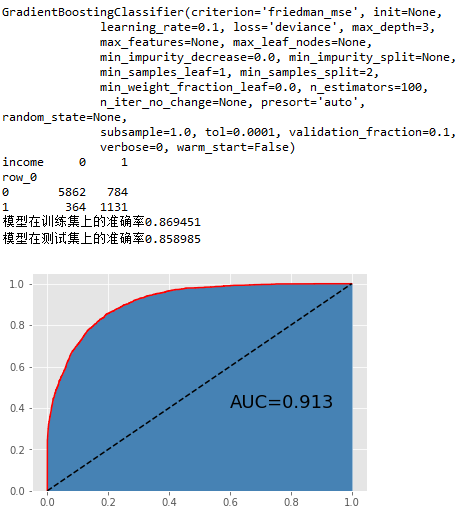# K近邻模型的网格搜索法
# 导入网格搜索法的函数
from sklearn.model_selection import GridSearchCV
# 选择不同的参数
k_options = list(range(1,12))
parameters = {'n_neighbors':k_options}
# 搜索不同的K值
grid_kn = GridSearchCV(estimator = KNeighborsClassifier(), param_grid = parameters, cv=10, scoring='accuracy', verbose=0)
grid_kn.fit(X_train, y_train)
print(grid_kn)
# 结果输出
print(grid_kn.grid_scores_, grid_kn.best_params_, grid_kn.best_score_)

# 预测测试集
grid_kn_pred = grid_kn.predict(X_test)
print(pd.crosstab(grid_kn_pred, y_test))

# 模型得分
print('模型在训练集上的准确率%f' %grid_kn.score(X_train,y_train))
print('模型在测试集上的准确率%f' %grid_kn.score(X_test,y_test))

# 绘制ROC曲线
fpr, tpr, _ = metrics.roc_curve(y_test, grid_kn.predict_proba(X_test)[:,1])
plt.plot(fpr, tpr, linestyle = 'solid', color = 'red')
plt.stackplot(fpr, tpr, color = 'steelblue')
plt.plot([0,1],[0,1], linestyle = 'dashed', color = 'black')
plt.text(0.6,0.4,'AUC=%.3f' % metrics.auc(fpr,tpr), fontdict = dict(size = 18))
plt.show()

# GBDT模型的网格搜索法
# 选择不同的参数
learning_rate_options = [0.01,0.05,0.1]
max_depth_options = [3,5,7,9]
n_estimators_options = [100,300,500]
parameters = {'learning_rate':learning_rate_options,'max_depth':max_depth_options,'n_estimators':n_estimators_options}

grid_gbdt = GridSearchCV(estimator = GradientBoostingClassifier(), param_grid = parameters, cv=10, scoring='accuracy')
grid_gbdt.fit(X_train, y_train)

# 结果输出
grid_gbdt.grid_scores_, grid_gbdt.best_params_, grid_gbdt.best_score_

# 预测测试集
grid_gbdt_pred = grid_gbdt.predict(X_test)
print(pd.crosstab(grid_gbdt_pred, y_test))

# 模型得分
print('模型在训练集上的准确率%f' %grid_gbdt.score(X_train,y_train))
print('模型在测试集上的准确率%f' %grid_gbdt.score(X_test,y_test))

# 绘制ROC曲线
fpr, tpr, _ = metrics.roc_curve(y_test, grid_gbdt.predict_proba(X_test)[:,1])
plt.plot(fpr, tpr, linestyle = 'solid', color = 'red')
plt.stackplot(fpr, tpr, color = 'steelblue')
plt.plot([0,1],[0,1], linestyle = 'dashed', color = 'black')
plt.text(0.6,0.4,'AUC=%.3f' % metrics.auc(fpr,tpr), fontdict = dict(size = 18))
plt.show()

注：来源刘顺祥《从零开始学Python数据分析与挖掘》，版权归原作者所有，仅供学习使用，不用于商业用途，如有侵权请留言联系删除，感谢合作。


展开全文• 快速了解数据分析与挖掘技术 1.什么是数据分析与挖掘技术（概念） 所谓数据分析，即对已知的数据进行分析，然后提取出一些有价值的信息，比如统计出平均数、标准差等信息，数据分析的数据量有时可能不会太大，而...
快速了解数据分析与挖掘技术

1.什么是数据分析与挖掘技术（概念）

所谓数据分析，即对已知的数据进行分析，然后提取出一些有价值的信息，比如统计出平均数、标准差等信息，数据分析的数据量有时可能不会太大，而数据挖掘，是指对大量的数据进行分析与挖掘，得到一些未知的，有价值的信息等，比如从网站的用户或用户行为数据中挖掘出用户的潜在需求信息，从而对网站进行改善。

数据分析与数据挖掘密不可分，数据挖掘是数据分析的提升。

2.数据分析与挖掘技术能做什么事情（概念 ）

数据挖掘技术可以帮助我们更好的发现事务之间的规律，所以，我们可以利用数据挖掘技术实现数据规律的探索、比如发现窃电用户、发掘用户潜在需求、实现信息的个性化推送、发现疾病与症状设置疾病与药物之间的规律...等

3.数据挖掘的过程主要有（怎么实现）

定义目标
获取数据（常用手段有通过爬虫采集或者下载一些统计网站发布的数据，并没有严格的数据把控）
数据探索（对我们数据的初步的研究，发现数据的特征，就知道我们接下来怎么处理）
数据预处理（数据清洗【去掉脏数据】、数据集成【集中】、数据变换【规范化】、数据规约【精简】）
挖掘建模（分类算法、聚类算法、关联算法、预测算法）
模型评价与发布
2.数据分析与挖掘相关模块简介与安装

1.相关模块简介

numpy可以高效处理数据、提供数组支持、很多模块都依赖他，比如pandas、scipy、matplotlib都依赖他，所以这个模块是基础。
pandas在后续用的最多的一个模块，主要用于进行数据探索和数据分析
matplotlib 作图模块，解决可视化问题
scipy 主要进行数值计算，同时支持矩阵运算，并提供了恩多高等数据处理功能，比如积分、傅里叶变换、微分方程求解等。
statsmodels这个模块主要用于统计分析
Gensim这模块主要用于文本挖掘
skearn、keras前者机器学习，后者深度学习
2.相关模块的安装与技巧（建议这样安装）

1.numpy、mkl（下载安装numpy+mkl） 提取码：n5ac  （可以自行选择安装版本numpy+mkl）

2.pandas （网络安装）

3.matplotlib （网络安装）

4.scipy（下载安装，网盘下载建议先下载在安装）提取码：i383   网站下载

5.statsmodels  （网络安装）

6.Gensim（网络安装）

3.相关模块的基本使用

1. numpy（数组的运算效率要比列表的运算效率要高，底层运算是不一样的）

1. #创建一维数组

>>> import numpy
>>> x = numpy.array(['a','v','b'])  #  创建数组的格式
>>> x
array(['a', 'v', 'b'], dtype='<U1')    # 返回对应的元素，和对应的类型，取值按照下标取，如：x  返回  v

#创建二维数组

>>> x = numpy.array([[4,5,6,7],[8,9,7,6],[12,23,34,8]])

>>> x

array([[ 4,  5,  6,  7],
[ 8,  9,  7,  6],
[12, 23, 34,  8]])   #返回数据

2.排序  sort()     按照上面例子如：x.sort()

3.取最大值和最小值

按照上面例子 ：取最大值 ：x.max()

取最小值： x.min()

4.切片

按照上面例子：x[起始下标：最终下标+1]

2.pandas使用

import pandas as pda
'''
Series   index索引未指定按照0开始    行列pandas里面的一维数组
DataFrame  表格行和列格式  二维数组
'''
a = pda.Series([8.9, 2, 1]) #索引未指定
b = pda.Series([8,9, 2, 1],index=["one", "two", "three", "four"])#指定索引，index必须和数据相对应

#第一种方法
data = pda.DataFrame([[54, 1, 21, 24], [7, 58, 88, 99], [47, 85, 98, 72], [69, 58, 47, 25]]) #默认行列以0开始
data = pda.DataFrame([[54, 1, 21, 24], [7, 58, 88, 99], [47, 85, 98, 72], [69, 58, 47, 25]],columns=['one','two','three','four']) #修改列名，必须跟数据长度一样
#第二种方法，按照字典创建  (one，two，three）为列表名
data_dict =pda.DataFrame({
'one':4,         一列一行
'two':[6,2,3],   value值是列表为多行，
'three':list(str(982))
})   #其他行不满足时，自动补全，如：

》》》   one  two three
0    4    6     9
1    4    2     8
2    4    3     2

b.tail()       #尾部数据，默认后五行
b.tail(行数)   #自定义取尾部数据几行
data.sort_values(,ascending=False) #排序，参数【0】为列名，ascending=False  降序

展示统计情况：
data.describe() #把数据的统计信息给打印出来
返回：
one        two      three       four
count   4.000000   4.000000   4.000000   4.000000  # 元素个数的信息
mean   44.250000  50.500000  63.500000  55.000000  # 代表的平均数
std    26.474831  35.369478  35.911929  36.905284  # 展示标准差的意思
min     7.000000   1.000000  21.000000  24.000000  # 这一列最小值
25%    37.000000  43.750000  40.500000  24.750000  # 代表每一列，分位数
50%    50.500000  58.000000  67.500000  48.500000  #
75%    57.750000  64.750000  90.500000  78.750000  #
max    69.000000  85.000000  98.000000  99.000000  # 这一列最大值

#数据转置（将行变成列，将列变成行）
data.T  #转置行遍列

3.matplotlib基础 折线图/散点图 以及绘制子图

# 折线图、散点图  一般会有 plot模块绘制
import matplotlib.pylab as pyl
import numpy as npy

# 维数x轴和y轴必须一致，否者报错
x = [1, 2, 3, 4, 8]  # x轴
y = [5, 7, 2, 1, 5]  # y轴
pyl.plot(x, y)  # plot(x轴，y轴，展现形式)一般会有两个或者3个参数，第一个x轴数据，第二是y轴参数，第三是对应展示的形式(如：或者展示为散点图，设置展示颜色 )
pyl.show()  # 展示折线图，默认折线图
pyl.plot(x, y, 'o')  # 字母o是代表散点图
pyl.show()  # 折线图如果不注释，将会和下面的散点图重叠展示
pyl.plot(x, y, 'c')  # 默认折线图，换成青色
pyl.plot(x, y, 'oc')  # 修改成散点图，换成青色，是否是折线图的参数和颜色的参数叠加在一起
pyl.show()
# 颜色代表
'''
c-cyan--青色
r-red--红色
m-magente-品红
g-green-绿色
b-blue-蓝色
y-yellow-huangs
k-black-黑色
w-white-白色
'''
# 线条样式 常用线条
'''
-    直线
--   虚线
-.-. 形式(展示就是-.形式)
:     细小虚线
'''
# pyl.plot(x, y, '--')  # 折线图修改线条
# 点的样式
'''
s   方形
h   六角形
H   六角形(跟h有一点区别)
*   星形
x   x形
d   菱形
D   菱形(与d有点区别)
p   五角星
'''
pyl.plot(x, y, "*")  # 加上点的样式，默认是散点图
pyl.show()
# 设置标题以及x轴与y轴名称
pyl.plot(x, y)
x2 = [1, 3, 6, 8, 10, 12, 19]
y2 = [1, 6, 9, 10, 19, 23, 35]
pyl.plot(x2, y2)
pyl.title('show')  # 标题
pyl.xlabel('ages')  # x轴名称
pyl.ylabel('temp')  # y轴名称
pyl.xlim(0, 20)  # 设置x轴的范围
pyl.ylim(1, 40)  # 设置y轴的范围
pyl.show()  # 使用多图绘制，在show之前，多次使用plot

随机数的生成
# 前提导入
import numpy as npy

# data = npy.random.random_integers(1, 20, 10)  # 生成整型数值，第一参数，最小值，第二参数：最大数，第三个参数：随机数的个数
# 怎么生成正太分布的随机数，生成正太分布使用normal模块
data=npy.random.normal(5.0,2.0,10)  # 均数，西格玛(越小越陡峭，越大越平缓)，个数
print(data)

# 随机数网址 http://www.mamicode.com/info-detail-507676.html
# 直方图  一般会有hist模块  绘制
# 直方图一般统计某一段数据的次数
#正太分布随机
data = npy.random.normal(10.0, 1.0, 10000)
pyl.hist(data)
pyl.show()
#整型随机
data1=npy.random.random_integers(1,25,1000)
pyl.hist(data1)
pyl.show()

# 直方图的宽度以及上下限
sty = npy.arange(2, 19, 2) #x轴开始和结束值，第三参数是数据范围条件
pyl.hist(data1,sty,histtype='stepfilled')#第二个参数不叫，显示默认的，histtype='stepfilled'取消直方图轮廓
pyl.show()

# 绘制子图
pyl.subplot(2, 2, 4)  # 参数1代表行，参数2代表列，参数3代表当前区域
pyl.show()
# 绘制两行一列

pyl.subplot(2, 2, 1)  # 共两行第一行分两列第一列
x1 = [1, 2, 3, 4, 5]
y1 = [5, 3, 5, 23, 5]
pyl.plot(x1, y1)

pyl.subplot(2, 2, 2)  # 共两行第一行分两列第2列
x2 = [5, 2, 3, 8, 6]
y2 = [7, 9, 12, 12, 3]
pyl.plot(x2, y2)

pyl.subplot(2, 1, 2)  # 共两行第二行分一列第2行
x3=[5,6,7,10,19,20,29]
y3=[6,2,4,21,5,1,5]
pyl.plot(x3,y3)
pyl.show()


展开全文• 《MATLAB数据分析与挖掘实战》文档pdf存于，D:\study\数据挖掘\MATLAB数据挖掘
《MATLAB数据分析与挖掘实战》文档pdf存于，D:\study\数据挖掘\MATLAB数据挖掘
展开全文• 前言 马云曾说“中国正迎来...这些数据就相当于隐藏在地球深处的宝贵资源，企业都想从数据红利中分得一杯羹，进而推进企业重视并善加利用数据分析与挖掘相关的技术。 本章将以概述的形式介绍数据分析挖掘相关的内...python 入门
• python数据分析与挖掘实战 pdf 张良均 资源共享 网盘下载： https://pan.baidu.com/s/1jQuthI-7NSDO5GWylu7YkQ 无提取码 永久有效
• 一直以来都有关注数据分析与挖掘领域，但网上信息繁杂，特此整理并筛选了python相关笔试题，供自己感兴趣的同仁共同学习提高。第一次发文，引陆游《冬夜读书示子聿 》的诗句共勉！ 古人学问无遗力，少壮工夫老始...大数据
• python数据挖掘项目实战记录取自《Python数据分析与挖掘实战》一书，整理各个项目中用到的数据处理方法： 数据预处理方法 建立模型方法 绘制图形 对于分类问题：用模型分类；混淆矩阵及其绘图；观测其ROC曲线； ...python
• 本文是基于《Python数据分析与挖掘实战》的实战部分的第15章的数据——《电商产品评论数据情感分析》做的分析。旨在回顾对评论文本数据的处理和建模方法。1 挖掘背景目标 对京东平台上的热水器评论进行文本挖掘...文本分析
• 本文是《Python数据分析与挖掘实战》的实战部分的第10章的数据——《家用电器用户行为分析事件识别》做的分析。 1.背景与挖掘目标 国内某智能热水器，在状态发生改变或有水流状态时会采集数据，该厂商根据采集到的...
• 本文是基于《Python数据分析与挖掘实战》的实战部分的第七章的数据——《航空公司客户价值分析》做的分析。旨在补充原文中的细节代码，并给出文中涉及到的内容的完整代码。 1）在数据预处理部分增加了属性规约、数据...K-means 航空公司客户价值分析 雷达图
• 数据分析涉及统计学、线性代数、图形分析绘制、数据挖掘等知识，推荐系统学习电子资料《利用Python进行数据分析第2版》、《Python数据分析与挖掘实战》、《从零开始学Python数据分析与挖掘》电子书和代码测试。...
• 更加挖掘目标和数据的形式可以建立分类预测、聚类分析、关联规则、时序模式和偏差检测等模式。 常见的分类预测算法： 回归分析： 回归分析是通过建立模型来演技变量之间相互关系的密切程度、结构状态及进行...算法 机器学习 python
• 经过10多个月的努力，《从零开始学Python--数据分析与挖掘》的新书上市啦，在此感谢清华大学出版社对本书提出的宝贵建议，也感谢广大网友及粉丝对我的期待。本书一共包含16章的内容，涉及四大模块，分别是Python基础...Python书籍
• 天善智能python3数据分析与挖掘实战视频教程 天善智能python3数据分析与挖掘实战视频教程 天善智能python3数据分析与挖掘实战视频教程 下载地址：百度网盘 ...
• 现整理python数据分析与挖掘相关面试题如下(代码已亲试)，供自己有需要的同仁共同学习提高。 活到老，学到老！终身学习！ 面试题 python数据分析 1 列举几个常用的python分析数据包及其作用 数据处理和分析：...面试题
• 本文是基于《Python数据分析与挖掘实战》的实战部分的第八章的数据——《中医证型关联规则挖掘》做的分析。旨在补充原文中的细节代码，并给出文中涉及到的内容的完整代码。主要有：1）将原始数据按照聚类结果进行...
• 转自：数据分析与挖掘的联系和区别！ 数据分析只是在已定的假设，先验约束上处理原有计算方法，统计方法，将数据分析转化为信息，而这些信息需要进一步的获得认知，转化为有效的预测和决策，这时...
• 本文是基于《Python数据分析与挖掘实战》的实战部分的第13章的数据——《财政收入影响因素分析及预测模型》做的分析。旨在补充原文中的细节代码，并给出文中涉及到的内容的完整代码。在作者所给代码的基础上增加的...
•python工程师 python爬虫 python python教程 python安装
• 本文是基于《Python数据分析与挖掘实战》的实战部分的第12章的数据——《电子商务网站用户行为分析及服务推荐》做的分析。旨在补充原文中的细节代码，并给出文中涉及到的内容的完整代码。在作者所给代码的基础上增加...
• 《Python数据分析与挖掘实战》这本书其实已经在暑假结束的时候就已经基本上过了一遍，但是却一直没有坚持着记录。最近几天想着将之前的学习内容整理一遍，因此，再做记录。 全文分为以下三个部分： Apriori算法 ...学习记录
• 《python数据分析与挖掘实战》（第2版）代码清单3.3的一点问题绘制频率分布直方图问题分析程序修改 绘制频率分布直方图 代码清单3.3的任务是根据“捞起生鱼片”的销售情况绘制频率分布直方图，其基本步骤如下： 求...python
• 问题今天看到《python数据分析与挖掘实战》这本书的第三章的第一份代码，照着书上的代码敲了一遍，发现在异常值处理的部分会报错。x = p['fliers'].get_xdata() y = p['fliers'].get_ydata()报错信息：...python 异常
• 本文是基于《Python数据分析与挖掘实战》的实战部分的第15章的数据——《电商产品评论数据情感分析》做的分析。 旨在回顾对评论文本数据的处理和建模方法。 1.挖掘背景目标 对京东平台上的热水器评论进行文本挖掘...
• 第一章－数据挖掘基础一、过程1、确定目标2、数据取样：随机取样、等距取样、分层取样、从起始顺序取样、分类抽样3、数据探索：异常值分析、缺失值分析、相关性分析和周期分析4、数据预处理：数据筛选、数据变量转换......